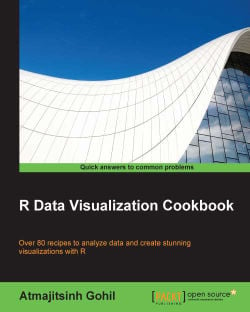# R Data Visualization Cookbook4.5 (4 reviews total)
By Atmajitsinh Gohil

• Constantly updated with 100+ new titles each month
• Breadth and depth in over 1,000+ technologies

R is an open source language for data analysis and graphics. It is platform-independent and allows users to load various packages as well as develop their own packages to interpret data better.

This book is packed with practical recipes, designed to provide you with all the guidance needed to get to grips with data visualization with R. It starts off with the basics of R plots and an introduction to heat maps and customizing them, before gradually taking you through creating interactive maps using the googleVis package, generating choropleth maps and contouring maps, bubble plots, and pie charts. You will then learn how to animate 2D and 3D plots in R. By the end of the book, you will be equipped with the key techniques to create impressive data visualizations with professional efficiency and precision.

Publication date:
January 2015
Publisher
Packt
Pages
236
ISBN
9781783989508

## Chapter 1. A Simple Guide to R

In this chapter, we will cover the following recipes:

• Installing packages and getting help in R

• Data types in R

• Special values in R

• Matrices in R

• Editing a matrix in R

• Data frames in R

• Editing a data frame in R

• Importing data in R

• Exporting data in R

• Writing a function in R

• Writing if else statements in R

• Basic loops in R

• Nested loops in R

• The apply, lapply, sapply, and tapply functions

• Using par to beautify a plot in R

• Saving plots

## Installing packages and getting help in R

If you are a new user and have never launched R, you must definitely start the learning process by understanding the use of `install.packages()`, `library()`, and getting help in R. R comes loaded with some basic packages, but the R community is rapidly growing and active R users are constantly developing new packages for R.

As you read through this cookbook, you will observe that we have used a lot of packages to create different visualizations. So the question now is, how do we know what packages are available in R? In order to keep myself up-to-date with all the changes that are happening in the R community, I diligently follow these blogs:

• Rblogger

• Rstudio blog

There are many blogs, websites, and posts that I will refer to as we go through the book. We can view a list of all the packages available in R by going to http://cran.r-project.org/, and also http://www.inside-r.org/packages provides a list as well as a short description of all the packages.

We can start by powering up our R studio, which is an Integrated Development Environment (IDE) for R. If you have not downloaded Rstudio, then I would highly recommend going to http://www.rstudio.com/ and downloading it.

### How to do it…

To install a package in R, we will use the `install.packages()` function. Once we install a package, we will have to load the package in our active R session; if not, we will get an error. The `library()` function allows us to load the package in R.

### How it works…

The `install.packages()` function comes with some additional arguments but, for the purpose of this book, we will only use the first argument, that is, the name of the package. We can also load multiple packages by using `install.packages(c("plotrix", "RColorBrewer"))`. The name of the package is the only argument we will use in the `library()` function. Note that you can only load one package at a time with the `library()` function unlike the `install.packages()` function.

### There's more…

It is hard to remember all the functions and their arguments in R, unless we use them all the time, and we are bound to get errors and warning messages. The best way to learn R is to use the active R community and the help manual available in R.

To understand any function in R or to learn about the various arguments, we can type `?<name of the function>`. For example, I can learn about all the arguments related to the `plot()` function by simply typing `?plot` or `?plot()` in the R console window. You will now view the help page on the right side of the screen. We can also learn more about the behavior of the function using some of the examples at the bottom of the help page.

If we are still unable to understand the function or its use and implementation, we could go to Google and type the question or use the Stack Overflow website. I am always able to resolve my errors by searching on the Internet. Remember, every problem has a solution, and the possibilities with R are endless.

## Data types in R

Everything in R is in the form of objects. Objects can be manipulated in R. Some of the common objects in R are numeric vectors, character vectors, complex vectors, logical vectors, and integer vectors.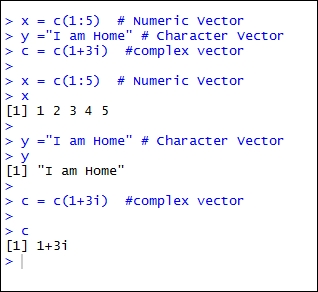### How to do it…

In order to generate a numeric vector in R, we can use the `C()` notation to specify it as follows:

`x = c(1:5) # Numeric Vector`

To generate a character vector, we can specify the same within quotes (" ") as follows:

`y ="I am Home" # Character Vector`

To generate a complex vector, we can use the `i` notation as follows:

`c = c(1+3i) #complex vector`

A list is a combination of a character and a numeric vector and can be specified using the `list()` notation:

`z = list(c(1:5),"I am Home") # List`

## Special values in R

R comes with some special values. Some of the special values in R are NA, Inf, -Inf, and NaN.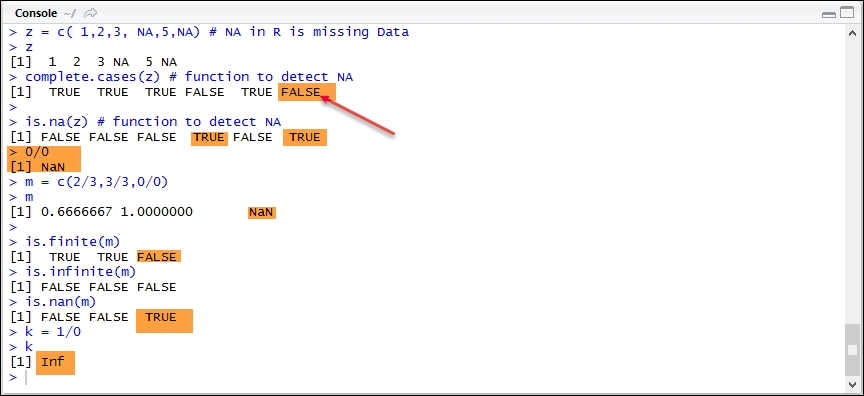### How to do it…

The missing values are represented in R by NA. When we download data, it may have missing data and this is represented in R by NA:

`z = c( 1,2,3, NA,5,NA) # NA in R is missing Data`

To detect missing values, we can use the `install.packages()` function or `is.na()`, as shown:

````complete.cases(z) # function to detect NA`
`is.na(z) # function to detect NA`
```

To remove the NA values from our data, we can type the following in our active R session console window:

```clean <- complete.cases(z)
z[clean] # used to remove NA from data```

Please note the use of square brackets (`[` `]`) instead of parentheses.

In R, not a number is abbreviated as NaN. The following lines will generate NaN values:

```##NaN
0/0
m <- c(2/3,3/3,0/0)
m```

The `is.finite`, `is.infinite`, or `is.nan` functions will generate logical values (`TRUE` or `FALSE`).

```is.finite(m)
is.infinite(m)
is.nan(m)```

The following line will generate `inf` as a special value in R:

```## infinite
k = 1/0```

### Tip

You can download the example code files for all Packt books you have purchased from your account at http://www.packtpub.com. If you purchased this book elsewhere, you can visit http://www.packtpub.com/support and register to have the files e-mailed directly to you.

### How it works…

`complete.cases(z)` is a logical vector indicating complete cases that have no missing value (NA). On the other hand, `is.na(z)` indicates which elements are missing. In both cases, the argument is our data, a vector, or a matrix.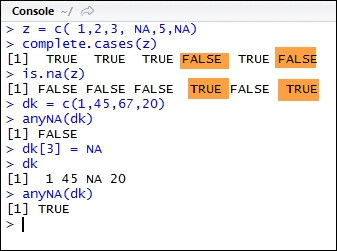R also allows its users to check if any element in a matrix or a vector is NA by using the `anyNA()` function. We can coerce or assign NA to any element of a vector using the square brackets ([ ]). The `` input instructs R to assign NA to the third element of the `dk` vector.

## Matrices in R

In this recipe, we will dive into R's capability with regard to matrices.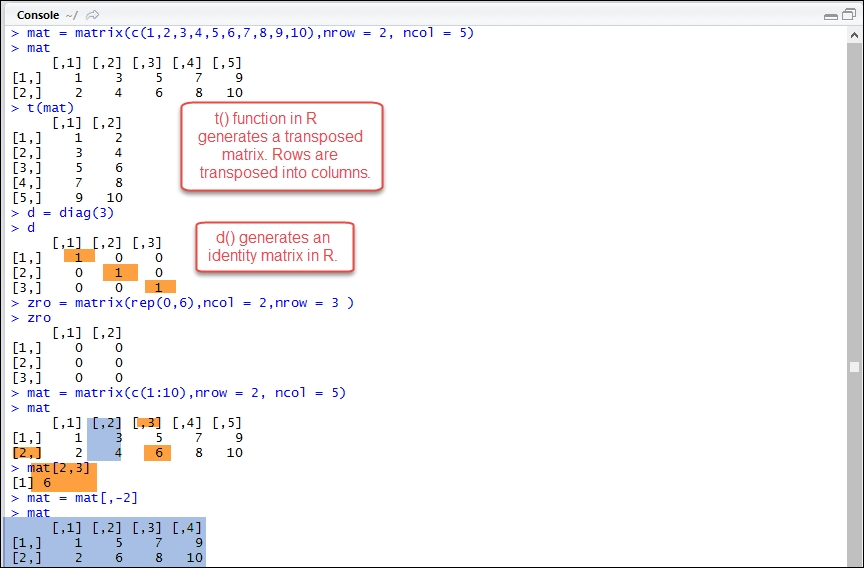### How to do it…

A vector in R is defined using the `c()` notation as follows:

`vec = c(1:10)`

A vector is a one-dimensional array. A matrix is a multidimensional array. We can define a matrix in R using the `matrix()` function. Alternatively, we can also coerce a set of values to be a matrix using the `as.matrix()` function:

```mat = matrix(c(1,2,3,4,5,6,7,8,9,10),nrow = 2, ncol = 5)
mat```

To generate a transpose of a matrix, we can use the `t()` function:

`t(mat) # transpose a matrix`

In R, we can also generate an identity matrix using the `diag()` function:

`d = diag(3) # generate an identity matrix`

We can nest the `rep ()` function within `matrix()` to generate a matrix with all zeroes as follows:

```zro = matrix(rep(0,6),ncol = 2,nrow = 3 )# generate a matrix of Zeros
zro```

### How it works…

We can define our data in the `matrix ()` function by specifying our data as its first argument. The `nrow` and `ncol` arguments are used to specify the number of rows and column in a matrix. The `matrix` function in R comes with other useful arguments and can be studied by typing `?matrix` in the R command window.

The `rep()` function nested in the `matrix()` function is used to repeat a particular value or character string a certain number of times.

The `diag()` function can be used to generate an identity matrix as well as extract the diagonal elements of a matrix. More uses of the `diag()` function can be explored by typing `?diag` in the R console window.

The code file provides a lot more functions that can used along with matrices—for example, functions related to finding a determinant or inverse of a matrix and matrix multiplication.

## Editing a matrix in R

R allows us to edit (add, delete, or replace) elements of a matrix using the square bracket notation, as depicted in the following lines of code:

```mat = matrix(c(1:10),nrow = 2, ncol = 5)
mat
mat[2,3]```

### How to do it…

In order to extract any element of a matrix, we can specify the position of that element in R using square brackets. For example, `mat[2,3]` will extract the element under the second row and the third column. The first numeric value corresponds to the row and the second numeric value corresponds to a column [row, column].

Similarly, to replace an element, we can type the following lines in R:

`mat[2,3] = 16`

To select all the elements of the second row, we can use `mat[2, ]`. If we do not specify any numeric value for a column, R will automatically assume all columns.

## Data frames in R

One of the useful and widely used functions in R is the `data.frame()` function. Data frame, according to the R manual, is a matrix structure whose columns can be of differing types, such as numeric, logical, factor, or character.

### How to do it…

A data frame in R is a collection of variables. A simple way to construct a data frame is using the `data.frame()` function in R:

```data = data.frame(x = c(1:4), y = c("tom","jerry","luke","brian"))
data```

Many times, we will encounter plotting functions that require data to be in a data frame. In order to coerce our data into a data frame, we can use the `data.frame()` function. In the following example, we create a matrix and convert it into a data frame:

```mat = matrix(c(1:10), nrow = 2, ncol = 5)
data.frame(mat)```

The `data.frame()` function comes with various arguments and can be explored by typing `?data.frame` in the R console window. The code file under the title `Data Frames – 2` provides additional functions that can help in understanding the underlying structure of our data. We can always get additional help by using the R documentation.

## Editing a data frame in R

Once we have generated a data and converted it into a data frame, we can edit any row or column of a data frame.

### How to do it...

We can add or extract any column of a data frame using the dollar (\$) symbol, as depicted in the following code:

```data = data.frame(x = c(1:4), y = c("tom","jerry","luke","brian"))
data\$age = c(2,2,3,5)
data```

In the preceding example, we have added a new column called `age` using the `\$` operator. Alternatively, we can also add columns and rows using the `rbind()` and `cbind()` functions in R as follows:

```age = c(2,2,3,5)
data = cbind(data, age)```

The `cbind` and `rbind` functions can also be used to add columns or rows to an existing matrix.

To remove a column or a row from a matrix or data frame, we can simply use the negative sign before the column or row to be deleted, as follows:

`data = data[,-2]`

The `data[,-2]` line will delete the second column from our data.

To re-order the columns of a data frame, we can type the following lines in the R command window:

```data = data.frame(x = c(1:4), y = c("tom","jerry","luke","brian"))
data = data[c(2,1)]# will reorder the columns
data```

To view the column names of a data frame, we can use the `names()` function:

`names(data)`

To rename our column names, we can use the `colnames()` function:

`colnames(data) = c("Number","Names")`

## Importing data in R

Data comes in various formats. Most of the data available online can be downloaded in the form of text documents (`.txt` extension) or as comma-separated values (`.csv`). We also encounter data in the tab-delimited format, XLS, HTML, JSON, XML, and so on. If you are interested in working with data, either in JSON or XML, refer to the recipe Constructing a bar plot using XML in R in Chapter 10, Creating Applications in R.

### How to do it...

In order to import a CSV file in R, we can use the `read.csv()` function:

`test = read.csv("raw.csv", sep = ",", header = TRUE)`

Alternatively, `read.table()` function allows us to import data with different separators and formats. Following are some of the methods used to import data in R: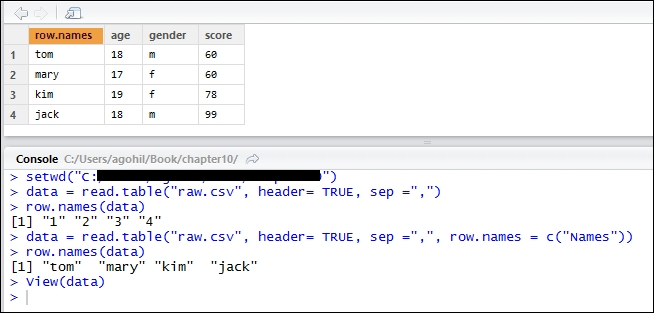### How it works…

The first argument in the `read.csv()` function is the filename, followed by the separator used in the file. The `header = TRUE` argument is used to instruct R that the file contains headers. Please note that R will search for this file in its current directory. We have to specify the directory containing the file using the `setwd()` function. Alternatively, we can navigate and set our working directory by navigating to Sessions | Set working directory | Choose directory.

The first argument in the `read.table()` function is the filename that contains the data, the second argument states that the data contains the header, and the third argument is related to the separator. If our data consists of a semi colon (;), a tab delimited, or the @ symbol as a separator, we can specify this under the `sep =""` argument. Note that, to specify a separator as a tab delimited, users would have to substitute `sep = ","` with `sep ="\t"` in the `read.table()` function.

One of the other useful arguments is the `row.names` argument. If we omit `row.names`, R will use the column serial numbers as `row.names`. We can assign `row.names` for our data by specifying it as `row.names = c("Name")`.

## Exporting data in R

Once we have processed our data, we need to save it to an external device or send it to our colleagues. It is possible to export data in R in many different formats.

### How to do it…

To export data from R, we can use the `write.table()` function. Please note that R will export the data to our current directory or the folder we have assigned using the `setwd()` function:

`write.table(data, "mydata.csv", sep=",")`

### How it works…

The first argument in the `write.table()` function is the data in R that we would like to export. The second argument is the name of the file. We can export data in the `.xls` or `.txt` format, simply by replacing the `mydata.csv` file extension with `mydata.txt` or `mydata.xls` in the `write.table()` function.

## Writing a function in R

Most of the tasks in R are performed using functions. A function in R has the same utility as functions in Arithmetic.

In order to write a simple function in R, we must first open a new R script by navigating to File | New file.

### How to do it…

We write a very simple function that accepts two values and adds them together. Copy and paste the code in the new blank R script:

```add = function (x,y){
x+y
}```

### How it works…

A function in R should be defined by `function()`. Once we define our function, we need to save it as a `.r` file. Note that the name of the file should be the same as the function; hence we save our function with name `add.r`.

In order to use the `add()` function in the R command window, we need to source the file by using the `source()` function as follows:

`source('<your path>/add.R')`

Now, we can type `add(2,15)` in the R command window. You get 17 printed as an output.

The function itself takes two arguments in our recipe but, in reality, it can take many arguments. Anything defined inside curly braces gets executed when we call `add()`. In our case, we request the user to input two variables, and the output is a simple sum.

• Functions can be helpful in performing repetitive tasks such as generating plots or perform complicated calculations. Felix Schönbrodt has implemented visually weighted watercolor plots in R using a function on his blog at http://www.nicebread.de/visually-weighted-watercolor-plots-new-variants-please-vote/.

• We can generate similar plots simply by copying the function created by Felix in our R session and executing it. The plotting function created by Felix also provides users with different ways in which the R function's ability could be leveraged to perform repetitive tasks.

## Writing if else statements in R

We often use `if` statements in MS Excel, but we can also write a small code to perform simple tasks in R.

### How to do it…

The logic for `if else` statements is very simple and is as follows:

```if(x>3){
print("greater value")
}else {
print("lesser value")
}```

We can copy and paste the preceding statement in the R console or write a function that makes use of the if else logic.

### How it works…

The logic behind `if` `else` statements is very simple. The following lines clearly state the logic:

```if(condition){
#perform some action
}else {
#perform some other action
}```

The preceding code will check whether x is greater than or less than 3, and simply print it. In order to get the value, we type the following in the R command window:

`x = 2`

## Basic loops in R

If we want to perform an action repeatedly in R, we can utilize the loop functionality.

### How to do it…

The following lines of code multiply each element of `x` and `y` and store them as a vector `z`:

```x = c(1:10)
y = c(1:10)
for(i in 1:10){
z[i] = x[i]*y[i]
}```

### How it works…

In the preceding code, a calculation is executed 10 times. R performs any calculation specified within `{}`. We are instructing R to multiply each element of `x` (using the `x[i]` notation) by each element in `y` and store the result in `z`.

## Nested loops in R

We can nest loops, as well as `if` statements, to perform some more complicated tasks. In this recipe, we will first define a square matrix and then write a nested for loop to print only those values where I = J, namely, the values in the matrix placed in (1,1), (2,2), and so on.

### How to do it…

We first define a matrix in R using the following `matrix()` function:

`mat= matrix(1:25, 5,5)`

Now, we use the following code to output only those elements where I = J:

```for (i in 1:5){
for (j in 1:5){
if (i ==j){
print(mat[i,j])
}
}
}```

The `if` statement is nested inside two `for` loop statements. As we have a matrix, we have to use two `for` loops instead of just one. The output of the matrix would be values such as 1, 7, 13, and 19.

## The apply, lapply, sapply, and tapply functions

R has some very handy functions such as `apply`, `sapply`, `tapply`, and `mapply`, that can be used to reduce the task of writing complicated statements. Also, using them makes our code look cleaner. The `apply()` function is similar to writing a loop statement.

The `lapply()` function is very similar to the `apply()` function but can be used on lists; this will return a list. The `sapply()` function is very similar to `lapply()` but returns a vector and not a list.

### How to do it…

The `apply()` function can be used as follows:

```mat= matrix(1:25, 5,5)
apply(mat,1,sd)```

The `lapply()` function can be used in the following way:

```j = list(x = 1:4, b = rnorm(100,1,2))
lapply(j,mean)```

The `tapply()` function is useful when we have broken a vector into factors, groups, or categories:

`tapply(mtcars\$mpg,mtcars\$gear,mean)`

### How it works…

The first argument in the `apply()` function is the data. The second argument takes two values: 1 and 2; if we state 1, R will perform a row-wise computation; if we mention 2, R will perform a column-wise computation. The third argument is the function. We would like to calculate the standard deviation of each row in R; hence we use the `sd` function as the third argument. Note that we can define our own function and replace it with the `sd` function.

With regard to the `lapply()` function, we have defined J as a list and would like to calculate the mean. The first argument in the `lapply()` function is the data and the second argument is the function used to process the data.

The first argument in the `tapply()` function is the data; in our case it is `mpg`. The second argument is the factor or the grouping; in this case it would be `gears`. The last argument is the function used to process the data. We would like to calculate the mean of `mpg` for each unique gear (3, 4, and 5 gears) in the mtcars data.

## Using par to beautify a plot in R

One quick and easy way to edit a plot is by generating the plot in R and then using Inkspace or any other software to edit it. We can save some valuable time if we know some basic edits that can be applied on a plot by setting them in a `par()` function. All the available options to edit a plot can be studied in detail by typing `?par` in the command window.

### How to do it…

In the following code, I have highlighted some commonly used parameters:

```x=c(1:10)
y=c(1:10)
par(bg = "#646989", las = 1, col.lab = "black", col.axis = "white",bty = "n",cex.axis = 0.9,cex.lab= 1.5)
plot(x,y, pch = 20, xlab = "fake x data", ylab = "fake y data")```

### How it works…

Under the `par()` function, we have set the background color using the `bg =` argument. The `las =` argument changes the orientation of the labels. The `col.lab` and `col.axis` arguments are used to specify the color of the labels as well as the axis. The `cex` argument is used to specify the size of the labels and axis. The `bty` argument is used to specify the box style in R.

## Saving plots

We can save a plot in various formats, such as `.jpeg`, `.svg`, `.pdf`, or `.png`. I prefer saving a plot as a `.png` file, as it is easier to edit a plot with Inkspace if saved in the PNG format.

### How to do it…

To save a plot in the .png format, we can use the `png()` function as follows:

```png("TEST.png", width = 300, height = 600)
plot(x,y, xlab = "x axis", ylab = "y axis", cex.lab = 3,col.lab = "red", main = "some data", cex.main=1.5, col.main = "red")
dev.off()```

### How it works…

We have used the `png()` function to save the plot as a PNG. To save a plot as a PDF, SVG, or JPEG, we can use the `pdf()`, `svg()`, or `jpeg()` functions, respectively.

The first argument in the `png()` function is the name of the file with the extension, followed by the width and height of the plot. We can now use the `plot()` function to generate a plot; any subsequent plots will also be saved with a `.png` extension, unless the `dev.off()` function is passed. The `dev.off()` function instructs R that we do not need to save the plots.

• ##### Atmajitsinh Gohil

Atmajitsinh Gohil works as a senior consultant at a consultancy firm in New York City. After graduating, he worked in the financial industry as a Fixed Income Analyst. He writes about data manipulation, data exploration, visualization, and basic R plotting functions on his blog at http://datavisualizationineconomics.blogspot.com.

He has a master's degree in financial economics from the State University of New York (SUNY), Buffalo. He also graduated with a master of arts degree in economics from University of Pune, India. He loves to read blogs on data visualization and loves to go out on hikes in his free time.

Browse publications by this author

### Latest Reviews

(4 reviews total)